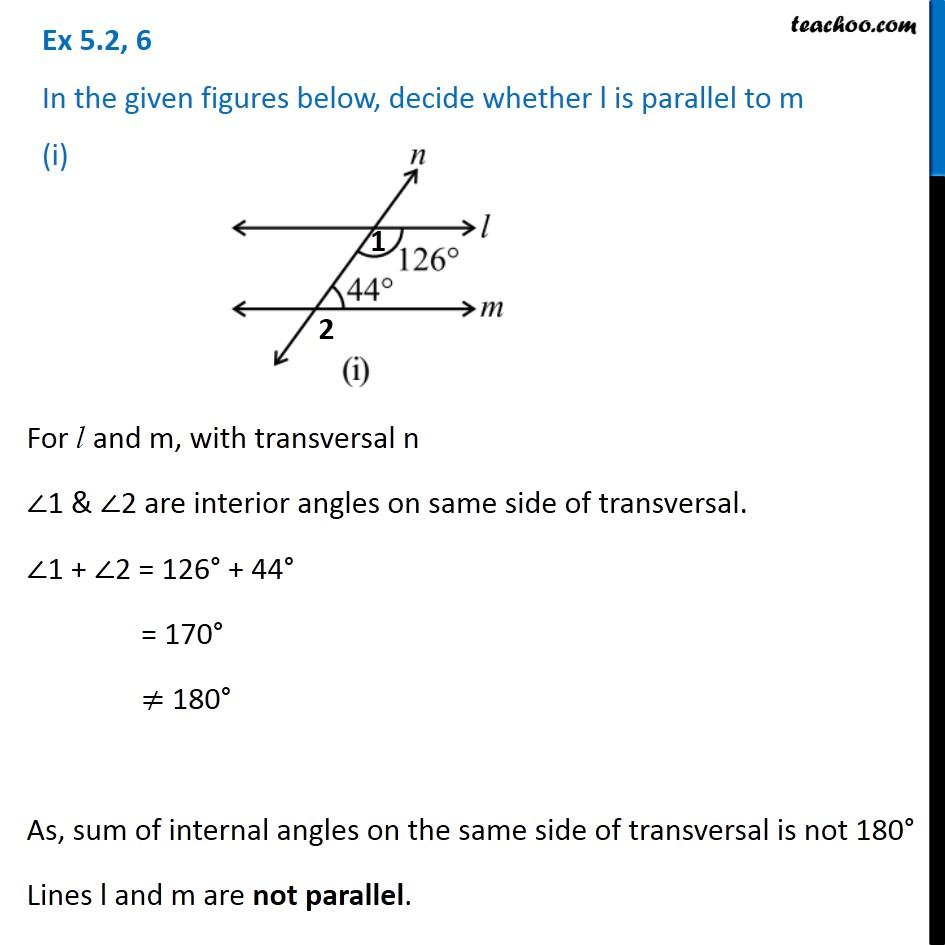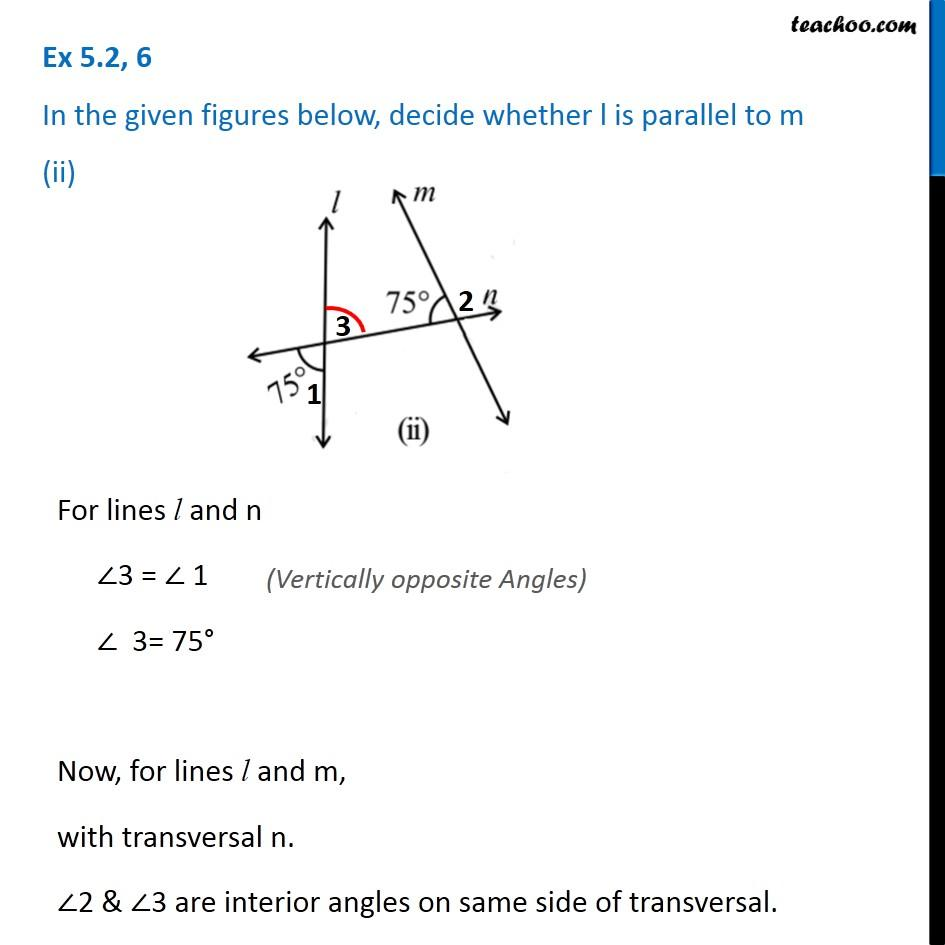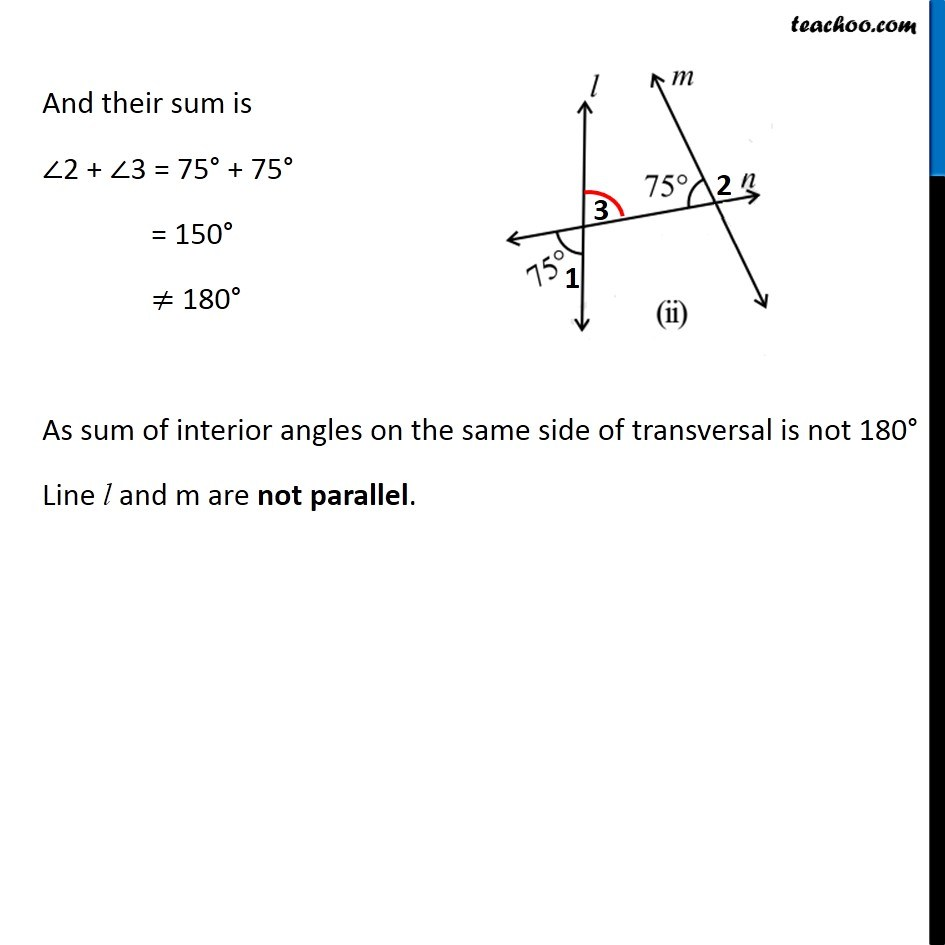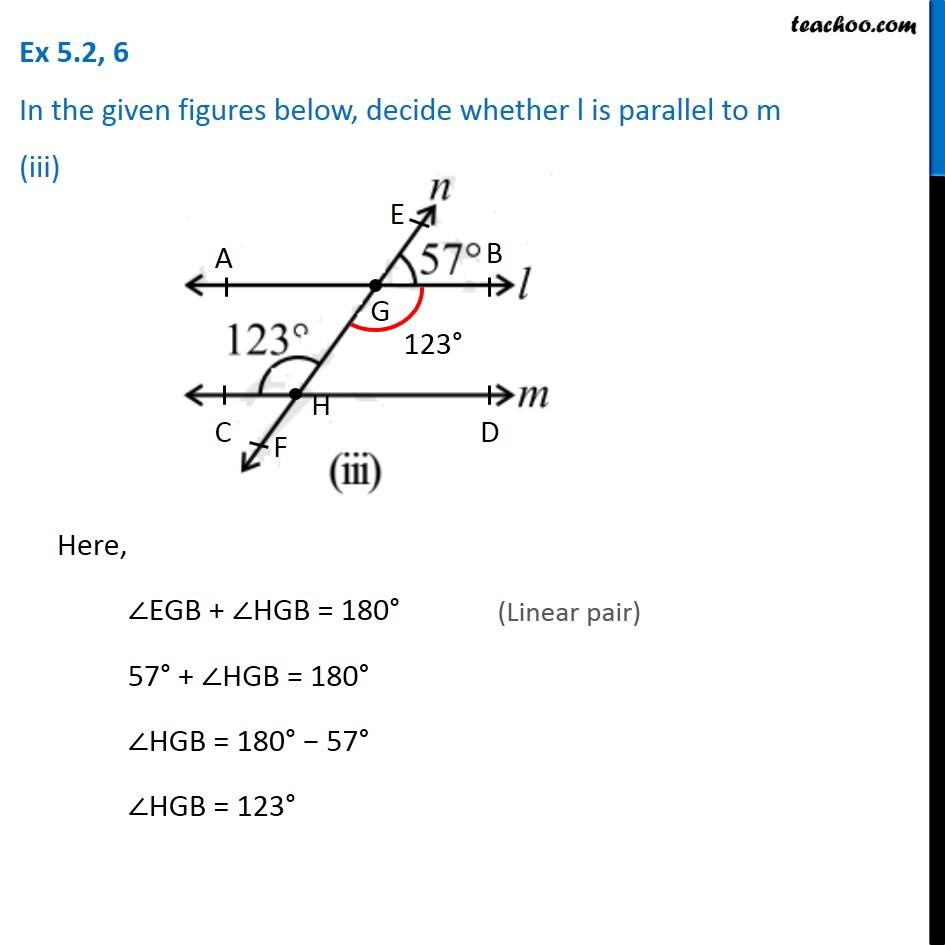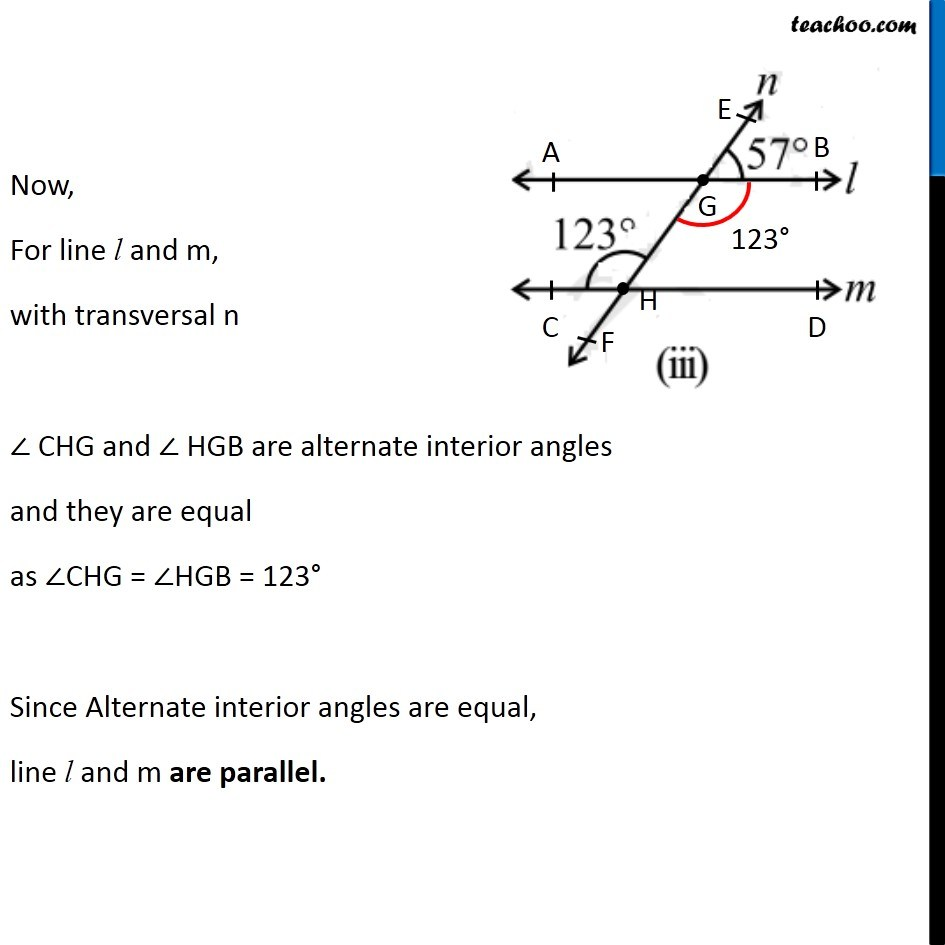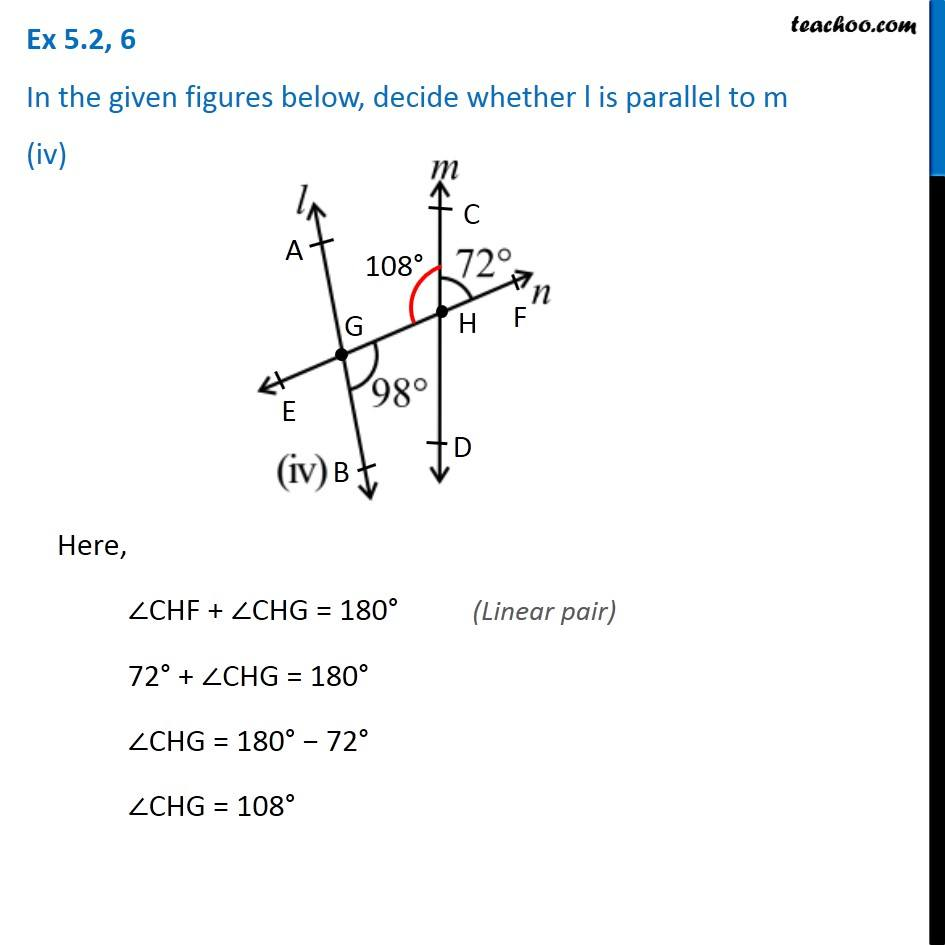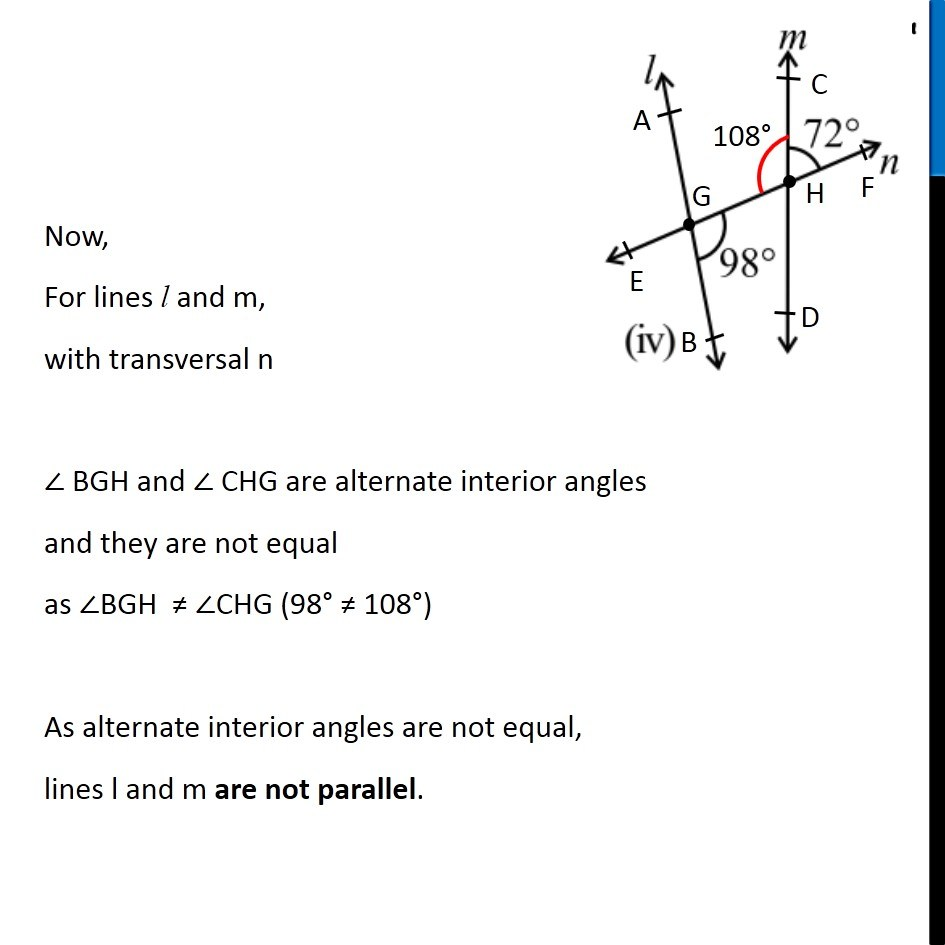Subscribe to our Youtube Channel - https://you.tube/teachoo

1. Chapter 5 Class 7 Lines and Angles
2. Serial order wise
3. Ex 5.2

Transcript

Ex 5.2, 6 In the given figures below, decide whether l is parallel to m (i)For l and m, with transversal n ∠1 & ∠2 are interior angles on same side of transversal. ∠1 + ∠2 = 126° + 44° = 170° ≠ 180° As, sum of internal angles on the same side of transversal is not 180° Lines l and m are not parallel. Ex 5.2, 6 In the given figures below, decide whether l is parallel to m (ii)For lines l and n ∠3 = ∠ 1 ∠ 3= 75° Now, for lines l and m, with transversal n. ∠2 & ∠3 are interior angles on same side of transversal. And their sum is ∠2 + ∠3 = 75° + 75° = 150° ≠ 180° As sum of interior angles on the same side of transversal is not 180° Line l and m are not parallel. Ex 5.2, 6 In the given figures below, decide whether l is parallel to m (iii) Here, ∠EGB + ∠HGB = 180° 57° + ∠HGB = 180° ∠HGB = 180° − 57° ∠HGB = 123° Now, For line l and m, with transversal n ∠ CHG and ∠ HGB are alternate interior angles and they are equal as ∠CHG = ∠HGB = 123° Since Alternate interior angles are equal, line l and m are parallel. Ex 5.2, 6 In the given figures below, decide whether l is parallel to m (iv) Here, ∠CHF + ∠CHG = 180° 72° + ∠CHG = 180° ∠CHG = 180° − 72° ∠CHG = 108° Now, For lines l and m, with transversal n ∠ BGH and ∠ CHG are alternate interior angles and they are not equal as ∠BGH ≠ ∠CHG (98° ≠ 108°) As alternate interior angles are not equal, lines l and m are not parallel.

Ex 5.2